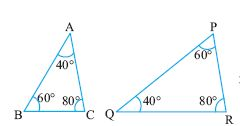Name: ___________________Date:___________________

 Email us to get an instant 20% discount on highly effective K-12 Math & English kwizNET Programs!

### Geometry2.23 Properties of Similar Triangles

 Congruency and Similarity: Figures that have same shape and size are said to be congruent, whereas figures need to be of the same shape but not necessarily of the same size for them to be similar. Properties of similar triangles Property 1: If in two triangles, angles are equal, then their corresponding sides are proportional and hence the triangles are similar. This property is called AAA postulate. Property 2: If one angle of two triangle is equal to the other and their sides are proportional, then the triangles are similar by SAS postulate. Property 3: If two angles and one side of two triangles are equal, then they are similar by ASA postulate. Example: In triangle ABC, prove that ABC and DEF are similar.Solution:: In triangles ABC and ADE, we have angle A = angle A (common angle) angle B = angle E (90o) Hence triangles ABC and ADE are similar. Directions: Solve the following problems. Also write at least 5 examples of your own.
 Q 1: State if the pair of triangles are congruent and if congruent by what postulate?Answer: Q 2: Find angle DCE80o45o30o Question 3: This question is available to subscribers only! Question 4: This question is available to subscribers only!